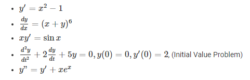# Ordinary Differential Equations

In mathematics, the term “Ordinary Differential Equations” also known as ODE is a relation that contains only one independent variable and one or more of its derivatives with respect to the variable. In other words, the ODE’S is represented as the relation having one real variable x, the real dependent variable y, with some of its derivatives.

Y’,y”, ….yn ,…with respect to x.

The order of ordinary differential equations is defined to be the order of the highest derivative that occurs in the equation. The general form of n-th order ODE is given as;

F(x, y,y’,….,yn ) = 0

Note that, y’ can be either dy/dx or dy/dt and yn can be either dny/dxn or dny/dtn.

An n-th order ordinary differential equations is linear if it can be written in the form;

a0(x)yn + a1(x)yn-1 +…..+ an(x)y = r(x)

The function aj(x), 0≤j≤n are called the coefficients of the linear equation. The equation is said to be homogeneous if r(x)=0.If r(x)≠0 , it is said to be a non- homogeneous equation. Also, learn the first order differential equation here.

## Ordinary Differential Equations Types

The ordinary differential equation is further classified into three types. They are:

• Autonomous ODE
• Linear ODE
• Non-linear ODE

### Autonomous Ordinary Differential Equations

A differential equation which does not depend on the variable, say x is known as an autonomous differential equation.

### Linear Ordinary Differential Equations

If differential equations can be written as the linear combinations of the derivatives of y, then it is known as linear ordinary differential equations. It is further classified into two types,

• Homogeneous linear differential equations
• Non-homogeneous linear differential equations

### Non-linear Ordinary Differential Equations

If differential equations cannot be written in the form of linear combinations of the derivatives of y, then it is known as non-linear ordinary differential equations.

## Ordinary Differential Equations Application

ODEs has remarkable applications and it has the ability to predict the world around us. It is used in a variety of disciplines like biology, economics, physics, chemistry and engineering. It helps to predict the exponential growth and decay, population and species growth. Some of the uses of ODEs are:

• Modelling the growth of diseases
• Describes the movement of electricity
• Describes the motion of the pendulum, waves
• Used in Newton’s second law of motion and Law of cooling.

### Ordinary Differential Equations Examples

Some of the examples of ODEs are as follows;### Ordinary Differential Equations Problems and Solutions

The ordinary differential equations solutions are found in an easy way with the help of integration. Go through once and get the knowledge of how to solve the problem.

Question 1:

Find the solution to the ordinary differential equation y’=2x+1

Solution:

Given, y’=2x+1

Now integrate on both sides, ∫ y’dx = ∫ (2x+1)dx

Which gives, y=2x2/2+x+c

y=x2+x+c

Where c is an arbitrary constant.

Question 2:

Solve y4y’+ y’+ x2 + 1 = 0

Solution:

Take, y’ as common,

y'(y4+1)=-x2-1

Now integrate on both sides, we get

$\frac{y^{5}}{5}+y=-\frac{x^{3}}{3}-x+c$

Where c is an arbitrary constant.

For more maths concepts, keep visiting BYJU’S and get various maths related videos to understand the concept in an easy and engaging way.